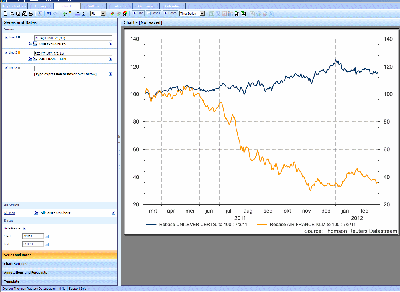## Stock Volatility and the P/H valueVolatility of stocks and indices has increased in importance the last few years. It is a measure to indicate the risk involved in investing in a stock or stock options. There are several methodes that indicate the volatility for individual stocks. One of these measures is the BETA of a stock. In previous blog posts I have shown how to get the BETA from Datastream using the BETA formula and how to use the formula for several stocks in one go.

Another method to get an indication of the volatility of a stock is the P/H value. The basis of this easy measure for volatility is the difference between the highest and lowest stock price over the last 12 months. The P/H value is calculated by dividing the difference of the highest and lowest price by the sum of these and multiplying this by the standard value 200. The formula looks as follows:

PH = ((PriceHighest – PriceLowest) / (PriceHighest + PriceLowest)) * 200

If the PH value is low then the stock price did not vary very much the last 12 months and has a low volatitlity. If the PH value is high, the stock has a high volatility and it may be riskier to invest in it (or in the stock options).

Here is an example of this I did in Datastream using the functions Max# and MIN# for Unilever and AIR/France KLM for the past year: 7-3-2011 to 7-3-2012. Below you see a screen-capture of the Time Series search I did using the Datastream AFO in Excel:The formulas (divided by a comma) in Datastream were:

MAX#(X(P),07/03/11,07/03/12)
MIN#(X(P),07/03/11,07/03/12)

The result of this search for both Unilever and AIR/France KLM is shown in Column C of the following screen-capture:The P/H value for Unilever is much lower than for AIR/France KLM. This shows that the stock of the latter was much more volatile over the last year. If I compare the rebased price for both stocks over the last year this is also immediately apparent:Email